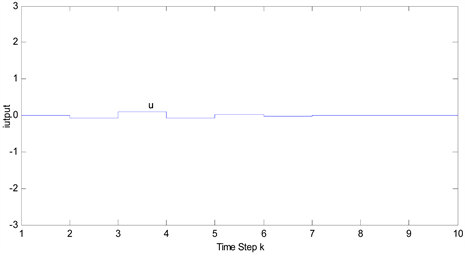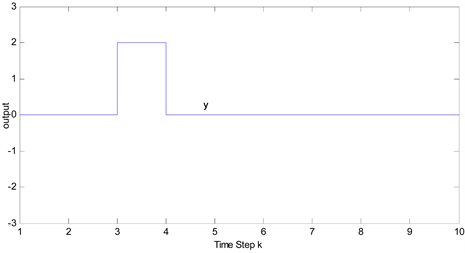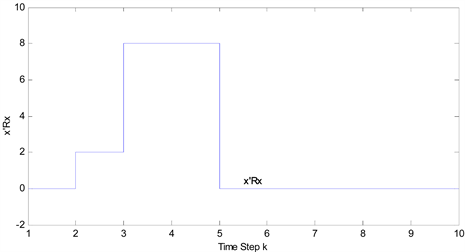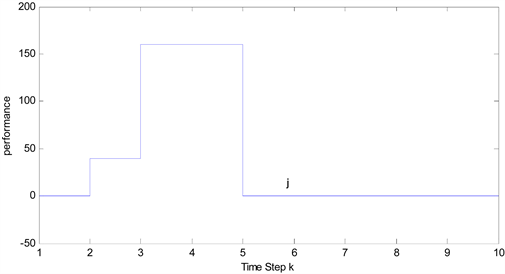﻿ 离散系统有限时间稳定预测控制

# 离散系统有限时间稳定预测控制Finite Time Stable Predictive Control for Discrete Systems

Abstract: The finite-time stable predictive control problem for a class of discrete-time linear time-invariant systems is studied. The concept of finite-time stability is introduced into predictive control, and the relationship between finite-time stability and predictive control is established by the state feedback. The minimization optimization problem in finite time domain is transformed into a constrained problem with linear matrix inequalities by constructing the Lyapunov function. The sufficient conditions for the existence of state feedback control rate are given by using linear matrix inequalities. The numerical simulation results show the effectiveness of the proposed method.

Abstract:

1. 引言

2. 问题描述

$\left\{\begin{array}{l}x\left(k+1\right)=Ax\left(k\right)+Bu\left(k\right),x\left(0\right)={x}_{0}\\ y\left(k+1\right)=Cx\left(k\right)\end{array}$ (1)

$\underset{u\left(k\right)}{\mathrm{min}}{J}_{N}\left(k\right)=\underset{u\left(k\right)}{\mathrm{min}}\underset{i=0}{\overset{N-1}{\sum }}{x}^{T}\left(k+i|k\right){Q}_{1}x\left(k+i|k\right)+{u}^{T}\left(k+i|k\right){Q}_{2}u\left(k+i|k\right)$ (2)

$u\left(k\right)=Kx\left(k\right)$ (3)

$x\left(k+1\right)=\left(A+BK\right)x\left(k\right)$ (4)

$V\left(x\left(k+i+1|k\right)\right)-V\left(x\left(k+i|k\right)\right)\le -\left({x}^{T}\left(k+i|k\right){Q}_{1}x\left(k+i|k\right)+{u}^{T}\left(k+i|k\right){Q}_{2}u\left(k+i|k\right)\right)$

${J}_{N}\left(k\right)\le V\left(x\left(k|k\right)\right)$ (5)

$x\left(k+1\right)=Ax\left(k\right),k\in {N}^{+}$ (6)

1) 系统(6)关于 $\left({\delta }_{x},\epsilon ,R,N\right)$ 是有限时间稳定的

2) 对于 $\forall k\in \left\{1,\cdots ,N\right\}$${\left({A}^{T}\right)}^{k}R{A}^{k}<\frac{{\epsilon }^{2}}{{\delta }_{x}^{2}}R$ 成立

3) 对于 $\forall k\in \left\{1,\cdots ,N\right\}$ ，若 ${P}_{k}\left(k\right)=R$${P}_{k}\left(h\right)={A}^{T}{P}_{k}\left(h+1\right)A,h\in \left\{0,1,\cdots ,k-1\right\}$

${P}_{k}\left(0\right)<\frac{{\epsilon }^{2}}{{\delta }_{x}^{2}}R$

4) 对于 $\forall k\in \left\{1,\cdots ,N\right\}$ ，存在对称矩阵值函数 ${P}_{k}\left(\cdot \right):h\in \left\{0,1,\cdots ,k\right\}↦{P}_{k}\left(h\right)\in {\Re }^{n×n}$

$S\left(x\right)=\left[\begin{array}{cc}{S}_{11}\left(x\right)& {S}_{12}\left(x\right)\\ {S}_{21}\left(x\right)& {S}_{22}\left(x\right)\end{array}\right]$ ，其中 ${S}_{11}\left(x\right)$ 是方阵。

1) $S\left(x\right)<0$

2) ${S}_{11}\left(x\right)<0$${S}_{22}\left(x\right)-{S}_{12}^{T}\left(x\right){S}_{11}^{-1}\left(x\right){S}_{12}\left(x\right)<0$

3) ${S}_{22}\left(x\right)<0$${S}_{11}\left(x\right)-{S}_{12}\left(x\right){S}_{22}^{-1}\left(x\right){S}_{12}^{T}\left(x\right)<0$

3. 有限时间稳定状态反馈

$\left(\begin{array}{cc}\begin{array}{l}-\gamma Q\\ AQ+BL\end{array}& \begin{array}{l}{\left(AQ+BL\right)}^{T}\\ -Q\end{array}\end{array}\right)<0$ (7)

$\frac{{\lambda }_{\mathrm{max}}\left(\stackrel{˜}{Q}\right)}{{\lambda }_{\mathrm{min}}\left(\stackrel{˜}{Q}\right)}<\frac{1}{{\gamma }^{N}}\frac{{\epsilon }^{2}}{{\delta }_{x}^{2}}$ (8)

$E={\left[{\left({C}_{1}A\right)}^{T}{\left({C}_{2}A\right)}^{T}\cdots {\left({C}_{r}A\right)}^{T}\right]}^{T}$

$S={\left[{S}_{1}^{T}{S}_{2}^{T}\cdots {S}_{r}^{T}\right]}^{T}$

${S}_{j}={C}_{j}B$$\left(j=1,\cdots ,r\right)$

$\stackrel{˜}{Q}={R}^{-\frac{1}{2}}Q{R}^{-\frac{1}{2}}$

$\begin{array}{c}{\gamma }^{k}V\left(x\left(0\right)\right)={\gamma }^{N}\left[x{\left(0\right)}^{T}Qx\left(0\right)\right]\\ \le {\gamma }^{N}\left[{\lambda }_{\mathrm{max}}\left(\stackrel{˜}{Q}\right)x{\left(0\right)}^{T}Rx\left(0\right)\right]\\ \le {\gamma }^{N}\left[{\lambda }_{\mathrm{max}}\left(\stackrel{˜}{Q}\right){\delta }_{x}^{2}\right]\end{array}$ (10)

$\begin{array}{c}V\left(x\left(k\right)\right)=x{\left(k\right)}^{T}Qx\left(k\right)\\ \ge {\lambda }_{\mathrm{min}}\left(\stackrel{˜}{Q}\right)x{\left(k\right)}^{T}Rx\left(k\right)\end{array}$ (11)

$x{\left(k\right)}^{T}Rx\left(k\right)\le \frac{{\lambda }_{\mathrm{max}}\left(\stackrel{˜}{Q}\right)}{{\lambda }_{\mathrm{min}}\left(\stackrel{˜}{Q}\right)}{\gamma }^{N}{\delta }_{x}^{2}<{\epsilon }^{2}$

$Q={P}^{-1}$ ，我们有 ${\stackrel{^}{A}}^{T}Q{}^{-1}\stackrel{^}{A}-\gamma {Q}^{-1}<0$

$\left(\begin{array}{cc}\begin{array}{l}-\gamma {Q}^{-1}\\ \stackrel{^}{A}\end{array}& \begin{array}{l}{\stackrel{^}{A}}^{T}\\ -Q\end{array}\end{array}\right)$

$\left(\begin{array}{cc}-\gamma Q& Q{\stackrel{^}{A}}^{T}\\ \stackrel{^}{A}Q& -Q\end{array}\right)=\left(\begin{array}{cc}-\gamma Q& Q{\stackrel{^}{A}}^{T}\\ \stackrel{^}{A}Q& -Q\end{array}\right)=\left(\begin{array}{cc}-\gamma Q& {\left(AQ+BKQ\right)}^{T}\\ \left(AQ+BKQ\right)& -Q\end{array}\right)$

$kQ=L$ ，同时 $Q={k}_{1}T$ ，此时有 $k=L{\left({k}_{1}T\right)}^{-1}$ ，即为所求的增益矩阵。

4. 仿真案例

$A=\left[\begin{array}{cccc}0& 1& 0& 0\\ -1& 0& 1& 0\\ 0& 0& 0& 1\\ 1& 0& -1& 0\end{array}\right]$$B=\left[\begin{array}{c}1\\ 0\\ 0\\ 0\end{array}\right]$$C=\left[\begin{array}{cccc}1& 0& 0& 0\end{array}\right]$Figure 1. States of the closed-loop systemFigure 2. The trajectory of the control inputFigure 3. Output of the closed-loop systemFigure 4. The trajectory of x’RxFigure 5. Performance index change

5. 总结

 Xue, W.P. and Mao, W.J. (2013) Asymptotic Stability and Finite-Time Stability of Networked Control Systems: Analysis and Syn-thesis. Asian Journal of Control, 15, 1376-1384.

 Dorato, P. (2005) An Overview of Finite-Time Stability. Current Trends in Nonlinear Systems and Control. Birkhauser Boston, 185-194.

 Haddad, W.M. and Lafflitto, A. (2015) Finite-Time Partial Stability and Stabilization and Optimal Feedback Control. Journal of the franklin Institute, 6, 2329-2357.
https://doi.org/10.1016/j.jfranklin.2015.03.022

 Amato, F., Ariola, M. and Cosentino, C. (2010) Finite-Time Control of Discrete-Time Linear Systems: Analysis and Design Conditions. Automatica, 46, 919-924.
https://doi.org/10.1016/j.automatica.2010.02.008

 Amato, F., Arioa, M. and Cosentino, C. (2001) Finite-Time Control of Linear Systems Subject to Parametric Uncertainties and Disturbances. Automatica, 37, 1459-1463.
https://doi.org/10.1016/S0005-1098(01)00087-5

 沈艳军. 一类线性离散时间系统有限时间控制问题[J]. 控制与决策, 2008(23): 107-109.

 Wang, L.M., Shen, Y. and Sheng, Y. (2016) Finite-Time Robust Stabilization of Uncertain Delayed Neural Networks with Discontinuous Activations via Delayed Feedback Control. The Official Journal of the International Neural Network Society, 76, 46-54.
https://doi.org/10.1016/j.neunet.2016.01.005

 严志国, 张国山. 线性随机系统有限时间 控制[J]. 控制与决策, 2011, 26(8): 1224-1228.

 Gao, M.Z., Cai, G.P. and Nan, Y. (2016) Finite Time Fault-Tolerant Control for Flutter of Wing. Control Engineering Practice, 51, 26-47.
https://doi.org/10.1016/j.conengprac.2016.03.007

 Gao, W.H., Deng, F.Q., Zhang, R.Q. (2014) Finite-Time Control for Time-Delayed Stochastic Systems with Markovian Switching. Hindawi Publishing Corporation, 1-10.

 席裕庚, 耿晓军, 陈虹. 预测控制性能研究的新进展[J]. 控制理论与应用, 2000, 17(4): 469-475.

 Amato, F. and Ariola, M. (2005) Finite-Time Control of Discrete-Time Linear Systems. IEEE Transactions on Auto-matic Control, 50, 724-729.
https://doi.org/10.1109/TAC.2005.847042

 Yza, E.E. (1998) Linear Maxtrix Inequalities in System and Control Theory. Proceeding of the IEEE, 86, 2473-2474.
https://doi.org/10.1109/JPROC.1998.735454

 袁璞, 左信, 郑海涛. 状态反馈预估控制[J]. 自动化学报, 1993, 19(5): 569-576.

Top